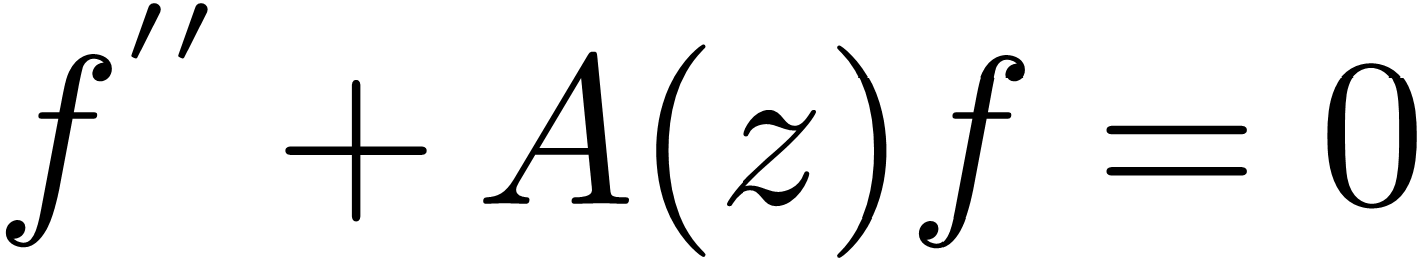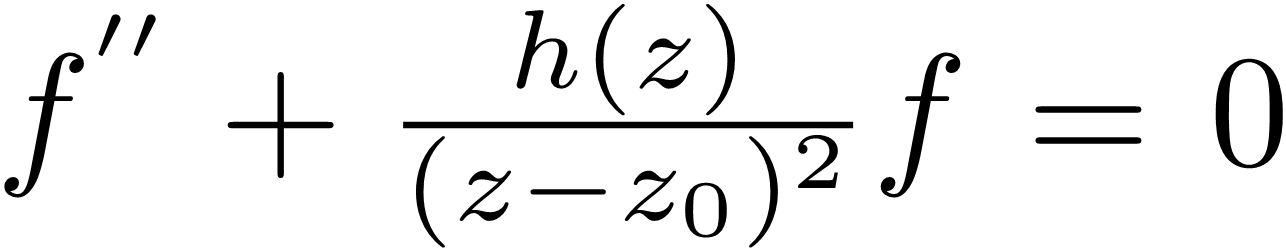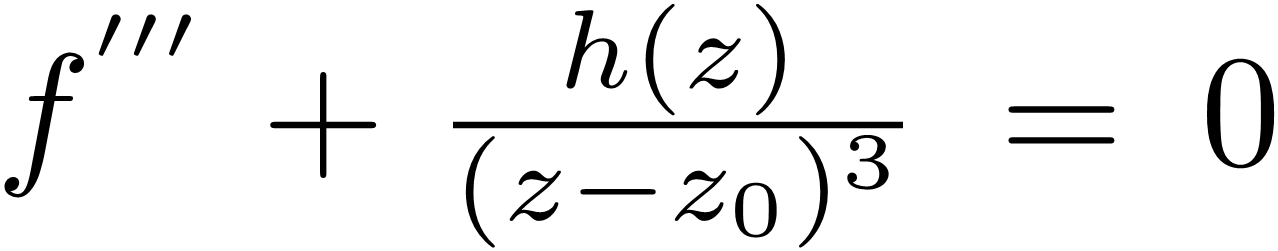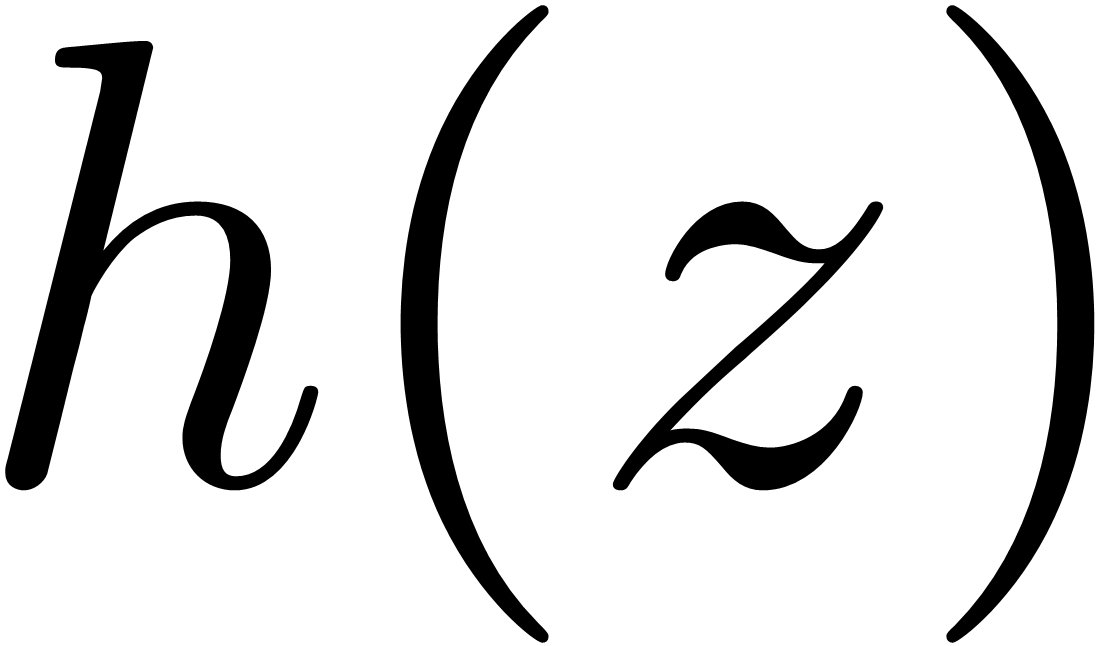Change code
ConfirmArchive
Special Issues
General Solutions of Some Complex Third-order Differential Equations
American Journal of Applied Mathematics
Volume 8, Issue 6, December 2020, Pages: 319-326
Received: Nov. 10, 2020; Accepted: Nov. 26, 2020; Published: Dec. 8, 2020
Authors
Rong Liao, School of Mathematical Sciences, South China Normal University, Guangzhou, China; Lihu Experimental School of Shenzhen University Town, Shenzhen, China
Zhibo Huang, School of Mathematical Sciences, South China Normal University, Guangzhou, China
Article ToolsAbstractPDF (299KB)
Abstract
According to the Nevanlinna theory, many researches have undertaken the behaviors of meromorphic solutions of complex ordinary differential equations (ODEs). Most of these researches have concentrated on the value distribution and growth of meromorphic solutions of ODEs. However, the existence of a meromorphic general solution is often used as a way to identify equations that are integrable. Especially, the existence of global meromorphic solutions of differential equationwith entire coefficient can be settled, resulting in the characterization of Schwarzian derivatives. This is concerning with the linearly independent solutions of linear differential equations. The purpose of this present paper is to find explicit solutions of differential equation in terms of finite combinations of known functions, that is, we use local series methods and reduction of order to solve all linearly independent solutions of some third-order ODEswith entire coefficientin the neighborhood of z0.
Keywords
Ordinary Differential Equation, Local Series Method, Linearly Independent, Meromorphic General Solution
Rong Liao, Zhibo Huang, General Solutions of Some Complex Third-order Differential Equations, American Journal of Applied Mathematics. Vol. 8, No. 6, 2020, pp. 319-326. doi: 10.11648/j.ajam.20200806.14
References

Ablowitz, M. J., and P. A. Clarkson. Soliton, nonlinear evolution equations and inverse scattering, London Mathematical Society Lecture Note Series, 149, Cambridge University Press, Cambridge, 1991.

Bank, S. B. Some results on analytic and meromorphic solutions of algebraic differential equations, Advances in Math., 15 (1975): 41–62.

Chiang, Y. M. and Halburd R. G., On the meromorphic solutions of an equation of Hayman, J.Math.Anal.Appl., 281 (2) (2003), 663–677.

Chen, W., Wang, Q. and Yuan, W., Meromorphic solutions of two certain types of nonlinear differential equations, Rocky Mountain J. Math., 50 (2) (2020), 479– 497.

Dilip Chandra, P., Jayuanta, R. and Kapil, R., On the growth of solutions of some non-linear complex differential equations, Korean J. Math., 28 (2) (2020), 295–309.

Gol’dberg, A. A. On single valued solution of first order differential equations (in Russian), Ukrain. Mat. Zh., 8, (1956), 254–261.

Halburd, R. and Korhonen, R., Growth of meromorphic solutions of delay differential equations, Proc. Amer. Math. Soc., 145 (6) (2017), 2513–2526.

Halburd, R. and Wang, J., All admissible meromorphic solutions of Hayman’s equation, Int. Math. Res. Not., 2015 (18) (2015), 8890–8902.

Hayman, W. K., The growth of solutions of algebraic differential equations, Mat. Appl., 7 (2) (1996), 67–73.

Hayman, W. K., Meromorphic Functions, Clarendon Press, Oxford, 1964.

Heittokangas, J., Ishizaki, K., Laine, I. andTohge, K., Complex oscillation and nonnscillation results, Trans. Amer. Math. Soc., 372 (9) (2019), 6161–6182.

Herold, H. Differentialglechungen im Komplexen, Vandenhoeck Ruprecht, Göttngen, 1975.

Laine, I., Nevanlinna theory and complex differental equations, de Gruyter, Berlin, 1993.

Long, J., Heittokangas, J., Ye, Z., On the relationship between the lower order of coefficients and the growth of solutions of differential equations, J. Math. Anal. Appl., 444 (1) (2016), 153–166.

Lü, F., Lü, W., Li, C. and Xu, J., Growth and uniqueness related to complex differential and difference equations, Results Math., 74 (1) (2019), 1–18.

Matsuda, M. First order algebraic differential equations, Lecture Notes in Math.,804, Springer, Berln, 1980.

Mokhon’ko, A. Z., and Kolyasa, L. I., Some properties of meromorphic solutions of linear differential equation with meromorphic coefficients, Mat. Stud., 52 (2) (2019), 166–172.

Zhang, R. R. and Huang, Z. B., Entire solutions of delay differential equations of Malmquist type, J. Appl. Anal. Comput., 10 (5) (2020), 1720–1740.
PUBLICATION SERVICES